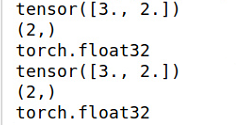﻿ pytorch关于Tensor的数据类型说明_python_脚本之家
python# pytorch关于Tensor的数据类型说明

## 关于Tensor的数据类型说明

1. 32位浮点型：torch.FloatTensor

```a=torch.Tensor( [[2,3],[4,8],[7,9]], )
print "a:",a
print "a.size():",a.size()
print "a.dtype:",a.dtype

b=torch.FloatTensor( [[2,3],[4,8],[7,9]] )
print "b:",b
print "b.shape:",b.shape
print "b.dtype:",b.dtype```2. 64位浮点型：torch.DoubleTensor

```b=torch.DoubleTensor( [[2,3],[4,8],[7,9]] )
print "b:",b
print "b.shape:",b.shape
print "b.dtype:",b.dtype```3. 16位整型：torch.ShortTensor

```b=torch.ShortTensor( [[2,3],[4,8],[7,9]] )
print "b:",b
print "b.shape:",b.shape
print "b.dtype:",b.dtype```4.  32位整型：torch.IntTensor

```b=torch.IntTensor( [[2,3],[4,8],[7,9]] )
print "b:",b
print "b.shape:",b.shape
print "b.dtype:",b.dtype```5. 64位整型：torch.LongTensor

```b=torch.LongTensor( [[2,3],[4,8],[7,9]] )
print "b:",b
print "b.shape:",b.shape
print "b.dtype:",b.dtype```6. 快速创建Tensor

（1） torch.zeros()

```a=torch.zeros( size=(4,5),dtype=torch.float32 )
print a
print a.shape
print a.dtype```（2） torch.randn()

```a=torch.randn( size=(4,5),dtype=torch.float32 )
print a
print a.shape
print a.dtype```7. Tensor索引方式，参考numpy

8. Tensor和numpy数组转换：

（1） Tensor转numpy，

```a=torch.randn( size=(4,5),dtype=torch.float32 )
print a
print a.shape
print a.dtype

b= a.numpy()
print b
print b.shape
print b.dtype```（2） numpy转Tensor，

```a=np.random.randn(4,3)
print a
print a.shape
print a.dtype

b=torch.from_numpy( a )
print b
print b.shape
print b.dtype```9.更改Tensor的数据类型，

```a=torch.FloatTensor( (3,2) )
print a
print a.shape
print a.dtype

a.int()
print a
print a.shape
print a.dtype```10. GPU加速，如果pytorch支持GPU加速，可以加Tensor放到GPU执行，

```if torch.cuda.is_available():
a_cuda = a.cuda()```

## pytorch Tensor变形函数view(), resize(), reshape() 在不改变原tensor数据的情况下修改tensor的形状，前后要求元素总数一致，且前后tensor共享内存transpose()函数可以将指定的两个维度的元素进行转置，而permute()函数则可以按照给定的维度进行维度变换。expand()函数将size为1的维度复制扩展为指定大小，也可以使用expand_as()函数指定为示例Tensor的维度。### Tensor的排序与取极值

max()与min()函数则是沿着指定维度选择最大与最小元素，返回该元素及对应的索引位置。### Tensor与NumPy转换

Tensor与NumPy可以高效地进行转换，并且转换前后的变量共享内存。在进行PyTorch不支持的操作时，甚至可以曲线救国，将Tensor转换为NumPy类型，操作后再转为Tensor。